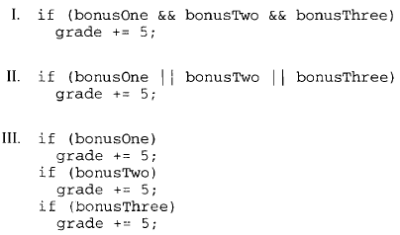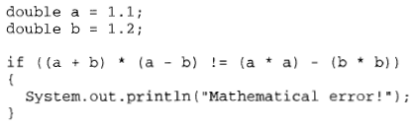# 2009 AP Computer Science A 计算机科学A真题系列之Released Exam选择题免费下载

### 2009 计算机科学A公开卷选择题部分免费下载

#### 部分真题预览：

2）A teacher put three bonus questions on a test and awarded 5 extra points to anyone who answered all three bonus questions correctly and no extra points otherwise. Assume that the boolean variables bonusOne, bonusTwo, and bonusThree indicate whether a student has answered the particular question correctly. Each variable was assigned true if the answer was correct and false if the answer was incorrect.Which of the follow code segments will properly update the variable grade based on a student's performance on the bonus questions?

1. I only
2. II only
3. III only
4. I and III
5. II and III

5)When designing a class hierarchy, which of the following should be true of a superclass?

1. A superclass should contain the data and functionality that are common to all subclasses that inherit from the superclass.
2. A superclass should be largest, most complex class from which all other subclasses are derived.
3. A superclass should contain the data and functionality that a re only required for the most complex class.
4. A superclass should have public data in order to provide access for the entire class hierarchy.
5. A superclass should contain the most specific details of the class hierarchy.

38)Consider the following segment.Which of the following best describes why the phrase "Mathematical error!" would be printed? (Remember that mathematically (a+b)*(a-b)= a2 - b2.)

1. Precedence rules make the if condition true.
2. Associativity rules make the if condition true.
3. Roundoff error makes the if condition true.
4. Overflow makes the if condition true.
5. A compiler bug or hardware error has occurred.

### 免费领取最新年份真题及解析